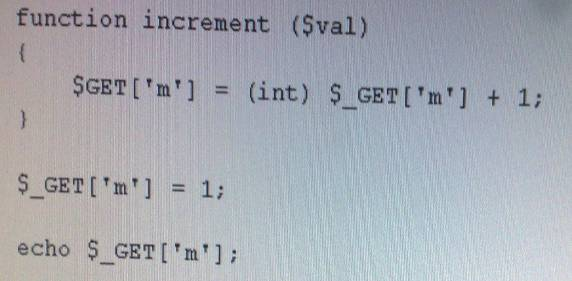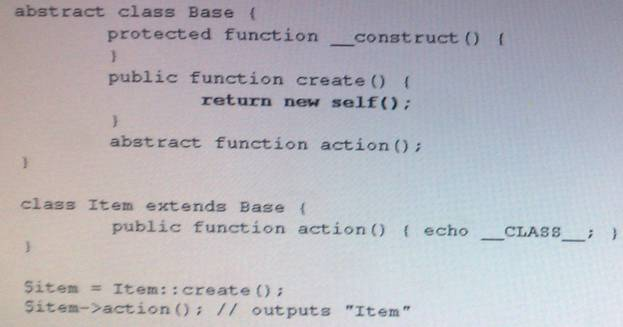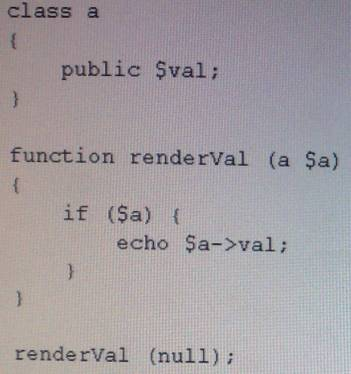# Zend PHP 5.3 Certification v6.1

Page:    1 / 17
Exam contains 259 questions

What function can reverse the order of values in an array without the loss of key information?

• A. array_flip()
• B. array_reverse()
• C. rsort()
• D. krsort()
• E. array_multisort()

What is the output of the following code?

• A. false-false
• B. false-true
• C. true-false
• D. true-true

Which of the following encryption standards provides symmetric key encryption? (Choose
2)

• A. AES
• B. Blowfish
• C. DES
• D. RSA

Webservices are primarily meant to support

• B. machine-to-machine interaction
• C. improved accessibility for websites

What do bytecode caches do?

• A. Cache the output of PHP scripts for reuse
• B. Translate PHP bytecode into machine code and cache the results
• C. Save the results of the compilation of PHP scripts for reuse
• D. Save large data structures (e.g. database result sets) in memory for reuse

What will be the output value of the following code?
\$array = array(1,2,3);
while (list(,\$v) = each(\$array));
var_dump(current(\$array));

• A. bool(false)
• B. int(3)
• C. int(1)
• D. NULL
• E. Array

What is the output of the following code?What is the output of the following code?

• A. An error
• B. 1,1,1,1,1,1,1,1,1,1,
• C. 1,1,2,3,5,8,13,21,34,55,
• D. Nothing

Consider the following code. Which keyword should be used in the line marked in bold to make this code work as intended?What is the output of the following code?• A. A syntax error in the function declaration line
• B. An error, because null is not an instance of 'a'
• C. Nothing, because a null value is being passed to renderVal()
• D. NULL

What is the result of the following code?

• A. Parse error
• B. 3.14
• C. PI
• D. T::PI

When retrieving data from URLs, what are valid ways to make sure all file_get_contents calls send a certain user agent string? (Choose 2)

• A. Option A
• B. Option B
• C. Option C
• D. Option D

In a shared hosting environment, session data can be read by PHP scripts written by any user. How can you prevent this?

• A. Store session data in a different location with session. save_Path .
• B. Store session data in a database.
• C. Enable safe_mode .
• D. Set session.name to something unique.

Which elements can be encapsulated by namespaces?

• A. Only classes
• B. Classes, functions and constants
• C. Classes, functions, constants and variables

What is the return value of the following code substr_compare("foobar", "bar", 3);

• A. -1
• B. 1
• C. TRUE
• D. 0
• E. FALSE